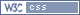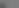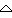Welcome, Guest. Please login or register. August 14, 2022, 05:17:44 AM 1 Hour 1 Day 1 Week 1 Month Forever Login with username, password and session length Forum changes: Editing of posts has been turned off until further notice.
 275647 Posts in 27717 Topics by 4285 Members Latest Member: - Jason DAngelo Most online today: 81 - most online ever: 565 (October 17, 2020, 02:08:06 PM)
 Pages: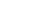Author Topic: Click-n-Locker  (Read 7091 times)
Grover
Member

Posts: 82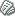« on: February 17, 2005, 08:36:45 PM »

I wrote up a Latex package to create Click-n-Locks.  There are 3 new commands:
\powers{Name}{Power1}{Power2}{Power3}{Power4}{Power5}{Style1}{Style3}{Style5}
\personalities{Name}{Attitude1}{Attitude2}{Attitude3}{Attitude4}{Attitude5}{Style2}{Style4}
\mundanes{Name}{Skill1}{Skill2}{Skill3}{Skill4}{Skill5}{Style1}{Style3}{Style5}

You don't get the neat pictures, and the fonts are kinda lousy, but they do fit together with the existing click-n-locks

Code:

capes.sty

\ProvidesPackage{capes}
\setlength{\unitlength}{1cm}
\newsavebox{\numberbox}
\savebox{\numberbox}(1,1)[lb]{
\put(0,0){\line(1,0){0.5}}
\put(0,0){\line(0,1){0.5}}
\put(0,0.5){\line(1,0){0.5}}
\put(0.5,0){\line(0,1){0.5}}
}
\newsavebox{\checkbox}
\savebox{\checkbox}(1,1)[lb]{
\put(0,0){\line(1,0){0.4}}
\put(0,0){\line(0,1){0.4}}
\put(0,0.4){\line(1,0){0.4}}
\put(0.4,0){\line(0,1){0.4}}
\put(0,0){\line(1,1){0.4}}
\put(0,0.4){\line(1,-1){0.4}}
}
\newcommand{\powers}{
\setlength{\unitlength}{1cm}
\begin{picture}(6.48,6)
\put(0,0.375){\line(0,1){4.01}}
\put(0.5,4.385){\oval(1.0,0.75)[lt]}
\put(0.5,4.76){\line(1,0){1}}
\put(3.75,4.76){\oval(4.5,0.75)}
\put(6.0,4.76){\line(1,0){5.13}}
\put(11.13,4.385){\oval(1.0,0.75)[rt]}
\put(11.63,4.183){\line(0,1){0.202}}
\put(11.13,4.183){\oval(1.0,0.75)[rb]}
\put(7.28,3.808){\line(1,0){3.85}}
\put(7.28,3.433){\oval(1.0,0.75)[lt]}
\put(6.78,3.231){\line(0,1){0.202}}
\put(7.28,3.231){\oval(1.0,0.75)[lb]}
\put(7.28,2.856){\line(1,0){3.85}}
\put(11.13,2.481){\oval(1.0,0.75)[rt]}
\put(11.63,2.279){\line(0,1){0.202}}
\put(11.13,2.279){\oval(1.0,0.75)[rb]}
\put(7.28,1.904){\line(1,0){3.85}}
\put(7.28,1.529){\oval(1.0,0.75)[lt]}
\put(6.78,1.327){\line(0,1){0.202}}
\put(7.28,1.327){\oval(1.0,0.75)[lb]}
\put(7.28,0.952){\line(1,0){3.85}}
\put(11.13,0.577){\oval(1.0,0.75)[rt]}
\put(11.63,0.375){\line(0,1){0.202}}
\put(11.13,0.375){\oval(1.0,0.75)[rb]}
\put(0.5,0){\line(1,0){10.63}}
\put(0.5,0.375){\oval(1.0,0.75)[lb]}
\put(1.5,4.61){\makebox[4.5cm][c]{#1}}
\put(6.78,4.134){\makebox[3.65cm][r]{#7}}
\put(6.78,2.23){\makebox[3.65cm][r]{#8}}
\put(6.78,0.326){\makebox[3.65cm][r]{#9}}
\put(10.63,4.034){\usebox{\numberbox}}
\put(10.63,2.13){\usebox{\numberbox}}
\put(10.63,0.226){\usebox{\numberbox}}
\put(0.5,3.7965){\makebox[5.38cm][r]{#2}}
\put(0.5,2.9195){\makebox[5.38cm][r]{#3}}
\put(0.5,2.0425){\makebox[5.38cm][r]{#4}}
\put(0.5,1.1655){\makebox[5.38cm][r]{#5}}
\put(0.5,0.2885){\makebox[5.38cm][r]{#6}}
\put(6.08,3.6965){\usebox{\numberbox}}
\put(6.08,2.8195){\usebox{\numberbox}}
\put(6.08,1.9425){\usebox{\numberbox}}
\put(6.08,1.0655){\usebox{\numberbox}}
\put(6.08,0.1885){\usebox{\numberbox}}
\end{picture}
}
\newcommand{\personalities}{
\setlength{\unitlength}{1cm}
\begin{picture}(12,6)
\put(0,3.231){\line(0,1){0.202}}
\put(0.5,3.433){\oval(1.0,0.75)[lt]}
\put(0.5,3.808){\line(1,0){3.85}}
\put(4.35,4.183){\oval(1.0,0.75)[rb]}
\put(4.85,4.183){\line(0,1){0.202}}
\put(5.35,4.385){\oval(1.0,0.75)[lt]}
\put(5.35,4.76){\line(1,0){1}}
\put(8.6,4.76){\oval(4.5,0.75)}
\put(10.85,4.76){\line(1,0){0.55}}
\put(11.4,4.385){\oval(1.0,0.75)[rt]}
\put(11.9,0.375){\line(0,1){4.01}}
\put(11.4,0.375){\oval(1.0,0.75)[rb]}
\put(5.35,0){\line(1,0){6.05}}
\put(5.35,0.375){\oval(1.0,0.75)[lb]}
\put(4.85,0.375){\line(0,1){0.202}}
\put(4.35,0.577){\oval(1.0,0.75)[rt]}
\put(0.5,0.952){\line(1,0){3.85}}
\put(0.5,1.327){\oval(1.0,0.75)[lb]}
\put(0,1.327){\line(0,1){0.202}}
\put(0.5,1.529){\oval(1.0,0.75)[lt]}
\put(0.5,1.904){\line(1,0){3.85}}
\put(4.35,2.279){\oval(1.0,0.75)[rb]}
\put(4.85,2.279){\line(0,1){0.202}}
\put(4.35,2.481){\oval(1.0,0.75)[rt]}
\put(0.5,2.856){\line(1,0){3.85}}
\put(0.5,3.231){\oval(1.0,0.75)[lb]}
\put(6.35,4.61){\makebox[4.5cm][c]{#1}}
\put(0.5,3.182){\makebox[3.05cm][r]{#7}}
\put(0.5,1.278){\makebox[3.05cm][r]{#8}}
\put(3.75,3.082){\usebox{\numberbox}}
\put(3.75,1.178){\usebox{\numberbox}}
\put(4.45,3.132){\usebox{\checkbox}}
\put(4.45,1.228){\usebox{\checkbox}}
\put(5.35,3.7965){\makebox[5.05cm][r]{#2}}
\put(5.35,2.9195){\makebox[5.05cm][r]{#3}}
\put(5.35,2.0425){\makebox[5.05cm][r]{#4}}
\put(5.35,1.1655){\makebox[5.05cm][r]{#5}}
\put(5.35,0.2885){\makebox[5.05cm][r]{#6}}
\put(10.6,3.6965){\usebox{\numberbox}}
\put(10.6,2.8195){\usebox{\numberbox}}
\put(10.6,1.9425){\usebox{\numberbox}}
\put(10.6,1.0655){\usebox{\numberbox}}
\put(10.6,0.1885){\usebox{\numberbox}}
\put(11.3,3.7465){\usebox{\checkbox}}
\put(11.3,2.8695){\usebox{\checkbox}}
\put(11.3,1.9925){\usebox{\checkbox}}
\put(11.3,1.1155){\usebox{\checkbox}}
\put(11.3,0.2385){\usebox{\checkbox}}
\end{picture}
}
\newcommand{\mundanes}{
\setlength{\unitlength}{1cm}
\begin{picture}(6.48,6)
\put(0,0.375){\line(0,1){4.01}}
\put(0.5,4.385){\oval(1.0,0.75)[lt]}
\put(0.5,4.76){\line(1,0){1}}
\put(3.75,4.76){\oval(4.5,0.75)}
\put(6.0,4.76){\line(1,0){5.13}}
\put(11.13,4.385){\oval(1.0,0.75)[rt]}
\put(11.63,4.183){\line(0,1){0.202}}
\put(11.13,4.183){\oval(1.0,0.75)[rb]}
\put(7.28,3.808){\line(1,0){3.85}}
\put(7.28,3.433){\oval(1.0,0.75)[lt]}
\put(6.78,3.231){\line(0,1){0.202}}
\put(7.28,3.231){\oval(1.0,0.75)[lb]}
\put(7.28,2.856){\line(1,0){3.85}}
\put(11.13,2.481){\oval(1.0,0.75)[rt]}
\put(11.63,2.279){\line(0,1){0.202}}
\put(11.13,2.279){\oval(1.0,0.75)[rb]}
\put(7.28,1.904){\line(1,0){3.85}}
\put(7.28,1.529){\oval(1.0,0.75)[lt]}
\put(6.78,1.327){\line(0,1){0.202}}
\put(7.28,1.327){\oval(1.0,0.75)[lb]}
\put(7.28,0.952){\line(1,0){3.85}}
\put(11.13,0.577){\oval(1.0,0.75)[rt]}
\put(11.63,0.375){\line(0,1){0.202}}
\put(11.13,0.375){\oval(1.0,0.75)[rb]}
\put(0.5,0){\line(1,0){10.63}}
\put(0.5,0.375){\oval(1.0,0.75)[lb]}
\put(1.5,4.61){\makebox[4.5cm][c]{#1}}
\put(6.78,4.134){\makebox[3.05cm][r]{#7}}
\put(6.78,2.23){\makebox[3.05cm][r]{#8}}
\put(6.78,0.326){\makebox[3.05cm][r]{#9}}
\put(10.03,4.034){\usebox{\numberbox}}
\put(10.03,2.13){\usebox{\numberbox}}
\put(10.03,0.226){\usebox{\numberbox}}
\put(10.73,4.084){\usebox{\checkbox}}
\put(10.73,2.18){\usebox{\checkbox}}
\put(10.73,0.276){\usebox{\checkbox}}
\put(0.5,3.7965){\makebox[4.78cm][r]{#2}}
\put(0.5,2.9195){\makebox[4.78cm][r]{#3}}
\put(0.5,2.0425){\makebox[4.78cm][r]{#4}}
\put(0.5,1.1655){\makebox[4.78cm][r]{#5}}
\put(0.5,0.2885){\makebox[4.78cm][r]{#6}}
\put(5.48,3.6965){\usebox{\numberbox}}
\put(5.48,2.8195){\usebox{\numberbox}}
\put(5.48,1.9425){\usebox{\numberbox}}
\put(5.48,1.0655){\usebox{\numberbox}}
\put(5.48,0.1885){\usebox{\numberbox}}
\put(6.18,3.7465){\usebox{\checkbox}}
\put(6.18,2.8695){\usebox{\checkbox}}
\put(6.18,1.9925){\usebox{\checkbox}}
\put(6.18,1.1155){\usebox{\checkbox}}
\put(6.18,0.2385){\usebox{\checkbox}}
\end{picture}
}Logged
TonyLB
Moderator
Member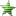Posts: 3702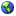« Reply #1 on: February 17, 2005, 09:16:54 PM »

Now that's extremely cool!  I have such fond memories of LaTeX from my college days....Logged

Just published: Capes
New Project:  Misery Bubblegum
Bret Gillan
Member

Posts: 375

That's Bret with one 't' damn it.« Reply #2 on: February 19, 2005, 12:05:50 PM »

I keep meaning to learn Latex. This gives me an added incentive. Thanks Grover, it looks really cool.Logged
MikeSands« Reply #3 on: February 20, 2005, 09:11:32 PM »

Tony,

Would it be possible for you to provide a pdf of blank click & locks on your website for those of us who don't want to go to the trouble of installing latex on our windows machines?Logged

TonyLB
Moderator
MemberPosts: 3702« Reply #4 on: February 20, 2005, 09:37:25 PM »

That's a darn fine idea, Mike.  Done.  The blank sheets are now in the downloads section, here.Logged

Just published: Capes
New Project:  Misery Bubblegum
MikeSands« Reply #5 on: February 20, 2005, 11:28:27 PM »

Thanks... I have to say, that's pretty darn good service!Logged

TonyLB
Moderator
MemberPosts: 3702« Reply #6 on: February 21, 2005, 06:19:49 AM »

I was up, it was easy.  Plus, I'm still feeling very guilty about the Inspiration Tracker sheet I need to write up, and the two-pages-to-a-letter-sized-page version of the PDF that I promised I'd look into.

Both of those projects are ongoing, but a bigger deal than the empty click-and-locks, so you get the quick thing as my guilt-payment in the meantime.Logged

Just published: Capes
New Project:  Misery Bubblegum
Christopher Weeks
Member

Posts: 683« Reply #7 on: February 21, 2005, 07:55:44 AM »

What would be really cool is a web application that lets you create customized Click-n-Lock pieces by typing in the powers and styles and whatnot and then draws an image that, when printed, fits the other Click-n-Locks.  :-)

edit: And while doing all this, it would database everything that anyone created so that your stock of support items for the game would grow automagically as we all used this tool.  That's actually a faintly brilliant tool for working with your fan community to help build your game up.Logged
TonyLB
Moderator
MemberPosts: 3702« Reply #8 on: February 21, 2005, 08:00:11 AM »

Yeah, that'd be really cool.  And I think I can do it, using Flash.  But I need to do some design work to get it up and running.

Y'know, maybe I should be creating a thread of "Things Tony is working on" combined with "Recommend things for Tony to work on".  I'm getting an awful lot of really good recommendations... ought to capitalize.Logged

Just published: Capes
New Project:  Misery Bubblegum
TonyLB
Moderator
MemberPosts: 3702« Reply #9 on: February 21, 2005, 01:54:03 PM »

Wow, that was easier than I thought it would be.

Power Locks
Skill Locks
Persona Locks

Fill in the abilities, then right-click anywhere that isn't a text field to get the Flash options menu, which will allow you to Print.

These are pretty rough and untested.  I think they should work, but if they go wrong for you I definitely want to hear it.

Having seen how easy this is, maybe I need to make a more comprehensive Flash tool... something that will let you:
[*]Create new modules[*]Print sheets of any modules you choose[*]Mix Power, Skill and Persona modules on the same page[*]Select modules from the book to print and use[*]Use modules (from the book or custom-created) to create characters on the character sheets.[/list:u]Not this weekend though.  Sorry.Logged

Just published: Capes
New Project:  Misery Bubblegum
John Harper« Reply #10 on: February 21, 2005, 05:13:39 PM »

Dude. You rule.Logged

Agon: An ancient Greek RPG. Prove the glory of your name!
Larry L.
Member

Posts: 616

aka Miskatonic« Reply #11 on: February 23, 2005, 01:34:46 PM »

Dude, you never told me you were a LaTeX whiz!

Hmm. Maybe I'll crank out a web-based perl/gd thingy.

If only we could convince Avery Dennison to release a line of pre-cut printer-ready label stock. Cutting out those "fingers" is a real drag.

Less cool looking, but easier to cut out would be something like:

Code:

********************* ==========
********************* ==========
********************* ==========
********** =====================
********** =====================Logged

Stickman
Member

Posts: 63« Reply #12 on: February 24, 2005, 12:42:36 AM »

I'd agree it looks less cool, but I think it has another advantage, that for powered clicks the powered styles are grouped together, making it a little easier to track debt producing actions.Logged

Dave
 Pages: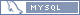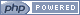Oxygen design by Bloc